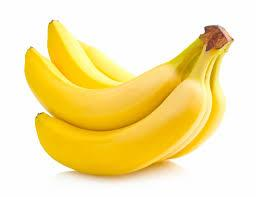# Disassembled 5815

The children disassembled 48 bananas on the trip. Some children took 1 banana each, 6 children took 2 bananas each, and last, Anina was the only one who did not take even 1 banana. How many children were on the trip?

n =  35

### Step-by-step explanation:

n = a+b+1
48 = a+2b
b= a-6

n = a+b+1
48 = a+2·b
b= a-6

a+b-n = -1
a+2b = 48
a-b = 6

Row 2 - Row 1 → Row 2
a+b-n = -1
b+n = 49
a-b = 6

Row 3 - Row 1 → Row 3
a+b-n = -1
b+n = 49
-2b+n = 7

Pivot: Row 2 ↔ Row 3
a+b-n = -1
-2b+n = 7
b+n = 49

Row 3 - 1/-2 · Row 2 → Row 3
a+b-n = -1
-2b+n = 7
1.5n = 52.5

n = 52.5/1.5 = 35
b = 7-n/-2 = 7-35/-2 = 14
a = -1-b+n = -1-14+35 = 20

a = 20
b = 14
n = 35

Our linear equations calculator calculates it.Did you find an error or inaccuracy? Feel free to write us. Thank you!

Tips for related online calculators
Do you have a system of equations and looking for calculator system of linear equations?